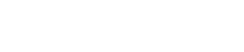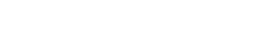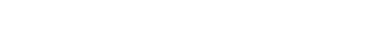AP Calculus Review: Disk and Washer Methods

The disk and washer methods are useful for finding volumes of solids of revolution. In this article, we’ll review the methods and work out a number of example problems. By the end, you’ll be prepared for any disk and washer methods problems you encounter on the AP Calculus AB/BC exam!

Solids of Revolution

The disk and washer methods are specialized tools for finding volumes of certain kinds of solids — solids of revolution. So what is a solid of revolution?

Starting with a flat region of the plane, generate the solid that would be “swept out” as that region revolves around a fixed axis.

For example, if you start with a right triangle, and then revolve it around a vertical axis through its upright leg, then you get a cone.The cone generated as a solid of revolution by revolving a right triangle around a vertical axis

Here’s another cool example of a solid of revolution that you might have seen hanging up as a decoration! Tissue paper decorations that unfold from flat to round are examples of solids of revolution. Watch the next few seconds of the video below to see how it unfolds in real time.

The Disk and Washer Methods: Formulas

So now that you know a bit more about solids of revolution, let’s talk about their volumes.

Suppose S is a solid of revolution generated by a region R in the plane. There are two related formulas, depending on how complicated the region R is.

Disk Method

The simplest case is when R is the area under a curve y = f(x) between x = a and x = b, revolved around the x-axis.Now imagine cutting the solid into thin slices perpendicular to the x-axis. Each slice looks like a disk or cylinder, except that the outer surface of the disk may have a curve or slant. Let’s approximate each slice by a cylinder of height dx, where dx is very small.

In fact, I like to think of each disk as being generated by revolving a thin rectangle around the x-axis. Then you can see that the height of the rectangle, y, is the same as the radius of the disk.

Now let’s compute the volume of a typical disk located at position x. The radius is y, which itself is just the function value at x. That is, r = y = f(x). The height of the disk is equal to dx (think of the disk as a cylinder standing on edge).

Therefore, the volume of a single cylindrical disk is: V = π r2h = π f(x)2dx.

This calculation gives the approximate volume of a thin slice of S. Next, to approximate the volume of the entirety of S, we have to add up all of the disk volumes throughout the solid. For simplicity, assume that the thickness of each slice is constant (dx). Also, for technical reasons, we have to keep track of the various x-values along the interval from a to b using the notation xk for a “generic” sample point.Finally, by letting the number of slices go to infinity (by taking a limit as n → ∞), we develop a useful formula for volume as an integral.Example 1: Disk Method

Let R be the region under the curve y = 2x3/2 between x = 0 and x = 4. Find the volume of the solid of revolution generated by revolving R around the x-axis.

Solution

Let’s set up the disk method for this problem.The volume of the solid is 256π (roughly 804.25) cubic units.

Washer Method

Now suppose the generating region R is bounded by two functions, y = f(x) on the top and y = g(x) on the bottom.

This time, when you revolve R around an axis, the slices perpendicular to that axis will look like washers.No, we’re not talking about clothes washers or dishwashers…

A washer is like a disk but with a center hole cut out. The formula for the volume of a washer requires both an inner radius r1 and outer radius r2.We’ll need to know the volume formula for a single washer.

V = π (r22r12) h = π (f(x)2g(x)2) dx.

As before, the exact volume formula arises from taking the limit as the number of slices becomes infinite.Example 2: Washer Method

Determine the volume of the solid. Here, the bounding curves for the generating region are outlined in red. The top curve is y = x and the bottom one is y = x2Solution

This is definitely a solid of revolution. We’ll set up the formula with f(x) = x (top) and g(x) = x2 (bottom). But what should we use as a and b?

Well, just as in some area problems, you may have to solve for the bounds. Clearly the region is bounded by the two curves between their common intersection points. Set f(x) equal to g(x) and solve to locate these points of intersection.

x = x2   →   xx2 = 0   →   x(1 – x) = 0.

We find two such points: x = 0 and 1. So set a = 0 and b = 1 in the formula.Example 3: Different Axes

Set up an integral that computes the volume of the solid generated by revolving he region bounded by the curves y = x2 and x = y3 around the line x = -1.

Solution

Be careful not to blindly apply the formula without analyzing the situation first!

This time, the axis of rotation is a vertical line x = -1 (rather than the horizontal x-axis). The radii will be horizontal segments, so think of x1 and x2 (rather than y-values).

Furthermore, because everything is turned on its side compared to previous problems, we have to make sure both boundary functions are solved for x. The thickness of the washer is now dy (instead of dx).

Finally, because the axis of revolution is one unit to the left of the y-axis, that adds another unit to each radius. (The further away the axis, the longer the radius must be to reach the figure, right?) Take a look at the graph below to help visualize what’s going on.

• Inner Radius: x = y3 + 1
• Outer Radius: x = y1/2 + 1As before, set the functions equal and solve for points of intersection. Those are again at x = 0 and 1.

Using the Washer Method formula for volume, we obtain:The problem only asks for setup, so we are done at this point.

Improve your SAT or ACT score, guaranteed. Start your 1 Week Free Trial of Magoosh SAT Prep or your 1 Week Free Trial of Magoosh ACT Prep today!Author

•Shaun earned his Ph. D. in mathematics from The Ohio State University in 2008 (Go Bucks!!). He received his BA in Mathematics with a minor in computer science from Oberlin College in 2002. In addition, Shaun earned a B. Mus. from the Oberlin Conservatory in the same year, with a major in music composition. Shaun still loves music -- almost as much as math! -- and he (thinks he) can play piano, guitar, and bass. Shaun has taught and tutored students in mathematics for about a decade, and hopes his experience can help you to succeed!